[BACK]
 Computers, Materials & ContinuaDOI:10.32604/cmc.2021.018709Article

Face Age Estimation Based on CSLBP and Lightweight Convolutional Neural Network

1School of Computer Science and Software Engineering, University of Science & Technology Liaoning, Anshan, 114051, China
2Medical and Health Sciences, University of Wollongong, NSW, 2522, Australia
*Corresponding Author: Ying Tian. Email: astianying@126.com
Received: 17 March 2021; Accepted: 18 April 2021

Abstract: As the use of facial attributes continues to expand, research into facial age estimation is also developing. Because face images are easily affected by factors including illumination and occlusion, the age estimation of faces is a challenging process. This paper proposes a face age estimation algorithm based on lightweight convolutional neural network in view of the complexity of the environment and the limitations of device computing ability. Improving face age estimation based on Soft Stagewise Regression Network (SSR-Net) and facial images, this paper employs the Center Symmetric Local Binary Pattern (CSLBP) method to obtain the feature image and then combines the face image and the feature image as network input data. Adding feature images to the convolutional neural network can improve the accuracy as well as increase the network model robustness. The experimental results on IMDB-WIKI and MORPH 2 datasets show that the lightweight convolutional neural network method proposed in this paper reduces model complexity and increases the accuracy of face age estimations.

Keywords: Face age estimation; lightweight convolutional neural network; CSLBP; SSR-Net

1  Introduction

In recent years, face age estimation has emerged as a popular research direction in the field of image processing and pattern recognition of facial attributes. Several techniques are available for face age estimation, and improved methods with higher accuracy have been applied. With the widespread application of mobile and light devices, facial age estimation algorithms must also adapt to this development trend and operate effectively on mobile terminals. However, mobile terminal equipment is affected by factors such as the complexity of the environment and the limitations of devices . In order to adapt to the limitations of the computing power and storage capacity of equipment, algorithms and those based on lightweight convolutional neural networks have been used. While applications play a significant role, the complexity of face images is also a major difficulty, predominantly due to the lack of image data of face images in the process of aging . The progression of human age is a slowly increasing process, and the appearance of human faces is highly varied. Facial images are also affected by real conditions such as illumination, facial posture, and occlusion, which increases the difficulty of the estimation of facial age.

The research and development of face age estimation can be simplified as the development of traditional artificial feature extraction combined with classifiers into deep learning technology for face age estimation. The use of face images for age estimation was first explored by Zhang et al. . They divided the face data set into three categories based on the geometric model of the face for the first time as children, adolescents, and adults. Their method employed the geometric proportions of the eyes, nose, and chin of the face as features for classification. Hammond et al.  used BP neural network as a classifier on the basis of several features to divide age into four stages: children, youth, middle-aged, and old. However, as the age of the geometric model of the face tended to stabilize, the applicability of the model decreased. Active Appearance Models (AAMs)  Aging Pattern Subspace (AGES) , manifold model , appearance model, etc.  have since made certain improvements. Ji et al.  applied the Local Binary Pattern (LBP) to face age estimation and achieved 80% accuracy on the FERET data set. Tang et al.  further improved the accuracy based on LBP descriptor and the AdaBoost algorithm.

As research into deep learning continues to expand and hardware conditions improve, research in the field of image classification has adopted deep learning for processing. Yang et al.  applied a multi-convolutional neural network to the estimation of age, gender, and race of face images. Yi et al.  proposed a deep sorting model using a multi-layer convolutional neural network for age estimation. Li et al.  proposed a multi-task model to perform multi-task parallel training on multiple attributes of the face and used WebFace and MORPH 2 data sets to experimentally prove the performance of the algorithm. As algorithms improve, network models have also developed in depth and breadth. After Xing et al.  applied AlexNet to image processing, deep learning developed rapidly in image processing. They subsequently proposed GoogLeNet  by increasing the network size while improving network performance. VGG  was then proposed, followed by ResNet . These convolutional neural networks extend the number of layers of the network to an unprecedented scale, and increase the complexity of the network while improving the accuracy, and are prone to overfitting. Under the real conditions that the high complexity of the network model is not suitable for mobile terminals, efforts are required to “reduce the burden” on the network model without reducing the accuracy as much as possible. Inception v3 was proposed in , which split two-dimensional convolution into one-dimensional convolution to reduce the number of parameters. SqueezeNet used 1 * 1 convolution instead of 3 * 3 convolution kernel to reduce the number of channels of output feature maps, while MobileNet reduced the number of network parameters while ensuring detection accuracy.

In order to adapt to the design and calculation capabilities of the mobile terminal, this paper proposes a lighter network than MobileNet to estimate facial age and uses Center Symmetric Local Binary Pattern (CSLBP) method to process the feature image. Face texture information is added to the original face image input by the network. The convolutional neural network not only automatically learns the original image information but also learns the texture information of the characteristic image, which improves the accuracy and robustness of the network model.

2  Related Work

2.1 Face Age Estimation

An abundant amount of information is recorded from facial recognition, including age, gender, identity, race, emotions, etc. . As a unique type of human information, age can not only effectively identify personal identity information but can also improve the stability and reliability of face recognition systems. Predicting the true (biological) age of a person from a face image is a classic problem in the field of computer vision and artificial intelligence . Face age estimation is suitable for monitoring, product recommendation, human-machine interfaces, commercial marketing, and other aspects . As of today, face age estimation remains a popular and challenging problem. The purpose of face age estimation is to calculate the age of a person from a picture or video of a given face area. The algorithm must learn a map from the facial picture or video to the age, using the correlation between the face area and estimated age. Employing the face area as an input makes the input state space of the algorithm huge. The complexity of a face picture, such as facial expression, face shape, makeup, and other factors, will have a visual impact, which is also related to the external environment, such as different lighting conditions, face angles, and visual qualities. Thus, when the face’s own attributes or external conditions change slightly, the prediction will be inaccurate.

2.2 Lightweight Convolutional Neural Network

The complexity of CNN continually increases as deep learning develops. Only by solving the problem of CNN efficiency can it move from the laboratory and be more widely used on mobile terminals . For efficiency issues, the usual method is to perform model compression, that is, to perform compression on the trained model so that the network carries fewer network parameters, thereby solving the memory and speed problems. Compared to processing on the already trained model, the lightweight model design is a different approach. The main idea of lightweight model design is to design a more efficient network calculation method (mainly for the convolution method) so that the network parameters are reduced without loss of network performance. Lightweight networks proposed in recent years mainly include MobileNet series (v1-v3), ShuffleNet series (v1-v2), SNet (the backbone of the lightweight target detection network ThunderNet, improved from shufflenetv2), and SSR-Net. The method proposed in this paper is to use SSR-Net’s lightweight convolutional neural network to realize face age estimation.

Soft Stagewise Regression Network is a new lightweight convolutional neural network model. The model adopts a strategy from coarse to fine and divides multiple classifications of age into multiple stages for execution. Each stage is only responsible for refining the decision of the previous stage to obtain a more accurate age estimate. This method greatly reduces the size of the model. At the same time, the model introduces dynamic range to solve the quantitative problem caused by age segmentation. Although the SSR-Net model is only 0.32 MB , it can achieve the comparable accuracy of a model 1500 times larger.

3  Face Age Estimation Based on CSLBP and Lightweight Convolutional Neural Network

In this paper, CSLBP is introduced on the original SSR-Net model to process the original input image in order to obtain a feature image with texture information. This is then combined with the original face image information as the input of the network and processed. However, as the difference between the original picture information and the feature map information is too large, the direct combination effect is not good. In order to make the original picture information consistent with the feature image information, the original picture is processed through a 1 × 1 convolution kernel for convolution operation, and then the convolutional feature map and the symmetry center local binary pattern information are combined as input information. The specific network structure is shown in Fig. 1 below.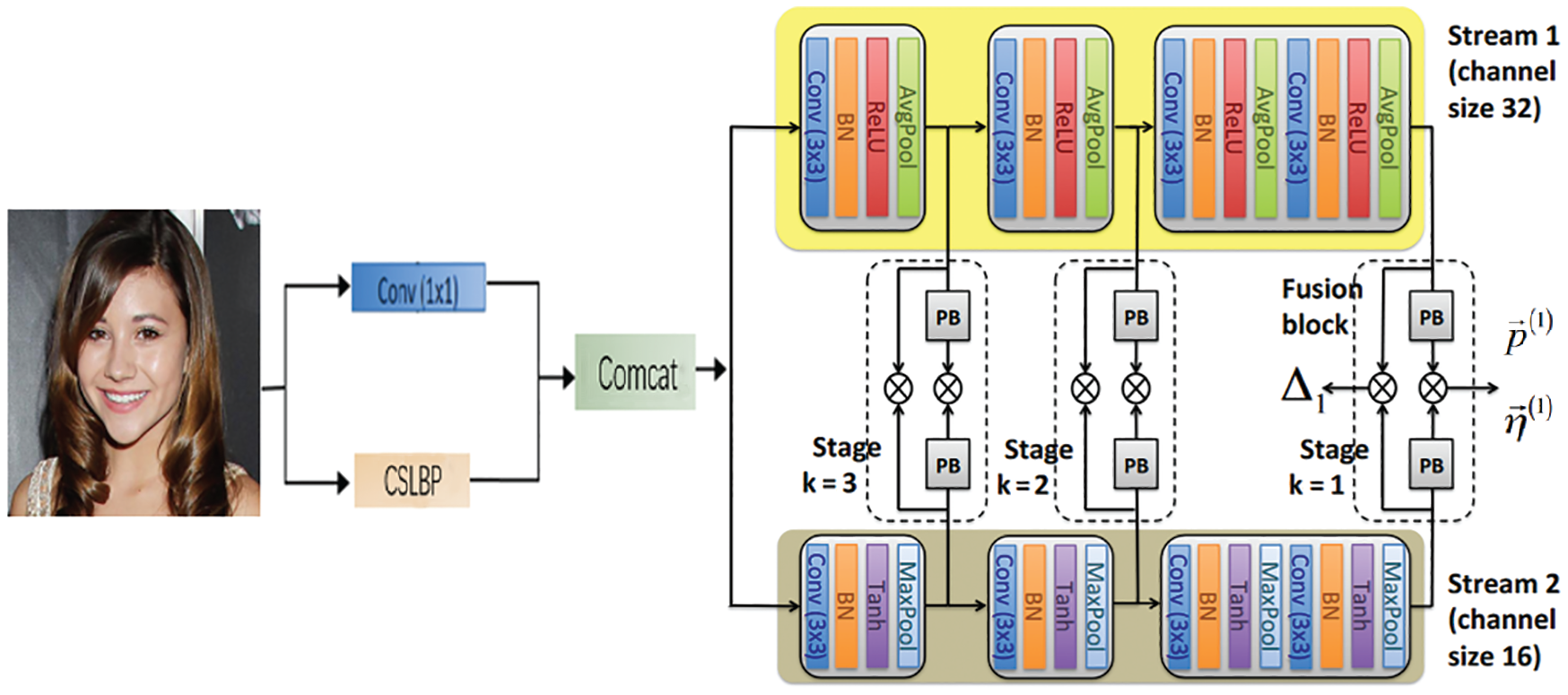Figure 1: Face age estimation model based on CSLBP and SSR-Net

The original face image is processed in two parts, one is to pass the input image through the convolutional layer, and the other is to obtain the feature image through the input image processed by CSLBP. Finally, feature fusion is performed through the ComCat layer, and the fused image is input into the network for facial age estimation.

3.1 CSLBP Description

Center Symm etric Local Binary Pattern (CSLBP)  is an improved algorithm based on local binary pattern (LBP) feature extraction. The traditional LBP operator eliminates the influence of light changes on the image to a certain extent . The impact on the image is also rotation invariant. The texture features extracted by LBP have a low latitude and are characterized by fast calculation speed. The idea of CSLBP is to introduce the central idea into the traditional LBP algorithm to encode and further improve on the traditional LBP operator. In the defined neighborhood, the CSLBP operator redefines the comparison rules between pixels; that is, only compares the pixel value pairs in the neighborhood with the center pixel as the center. If it is greater than or equal to the center pixel, it is 1; otherwise, it is 0. This provides an ordered binary string, which is then converted into a decimal number as the code of the center pixel. The principle of CSLBP is shown in Fig. 2.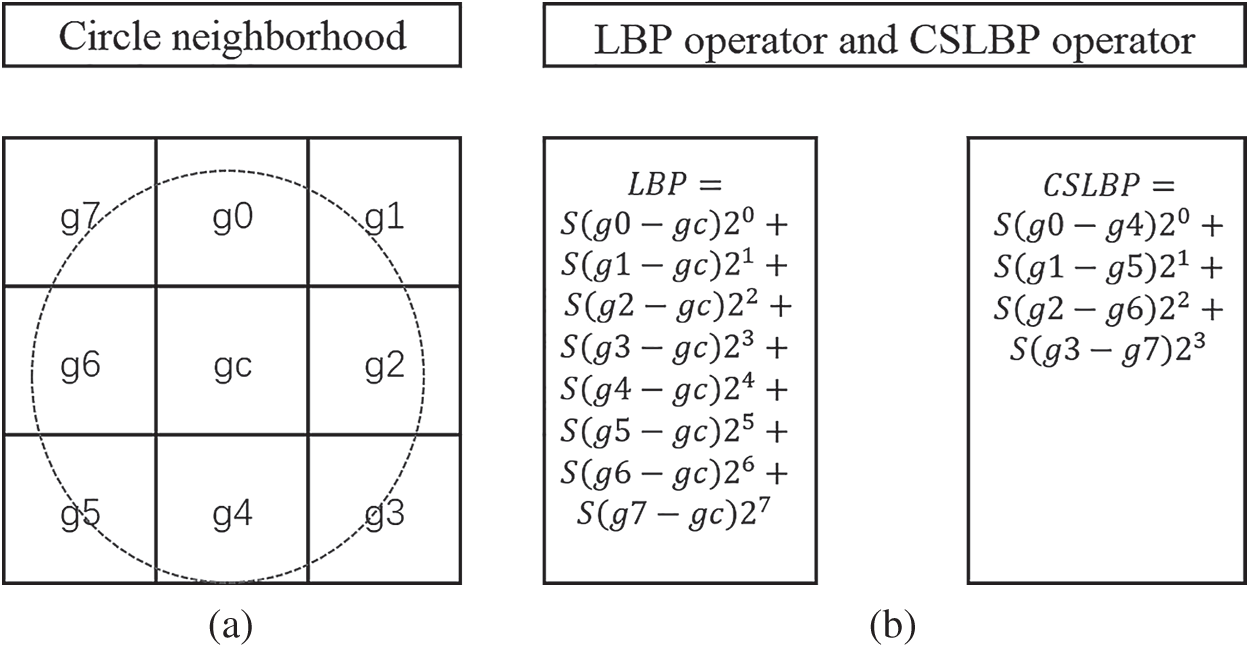Figure 2: Schematic diagram of CSLBP principle. (a) Schematic diagram of circle neighborhood (b) Comparing calculation between LBP operator and CSLBP operator

Compared with using the LBP operator alone, the CSLBP operator method can provide better image analysis results . For any given pixel position in an image, in addition to being able to indicate the relationship of the magnitude of the value of the surrounding pixel positions, it can also better describe the structural relationship between it and the surrounding pixels in the spatial position through the diagonal encoding method in the four main directions. As this method is highly tolerant to lighting changes and blurs and reduces the amount of calculation, it can enhance the anti-noise ability of the extracted features. In Fig. 2, image (a) represents the defined circle neighborhood (8, 1), and image (b) represents the LBP encoding and CSLBP operator of the center pixel gc of the neighborhood, respectively. The expressions defined by the encoding method are as follows:

CSLBP(P,R)(x,y)=i=0(N2)1S(gigi+(N2))2i (1)

LBP(P,R)(x,y)=i=0N1S(gigc)2i (2)

S(x)={1x00otherwise (3)

Eqs. (1) and (2) are the CSLBP operator and LBP operator, respectively, where (P, R) represents the circle neighborhood. Among them, P represents the number of pixels on the circle, R represents the circle radius, and N = P. In the same neighborhood, the LBP operator compares the center pixel value with other pixel values in the area and obtains a binary pattern string of length P, which is then converted into a decimal number; CSLBP compares the center pixel value to the center symmetric pixel value pairs, obtains a binary pattern string of length P/2, and then converts it to a decimal number. The above analysis shows that CSLBP and LBP have similar processing principles, but CSLBP has obvious advantages in extracting feature dimensions and storage space requirements. The decrease in computing feature dimensions greatly reduces the time spent on calculations and improves processing while effectively extracting features.

3.2 SSR-Net

SSR-Net is an improved network inspired by DEX. DEX solves the age estimation problem by performing multi-class classification and then converts the classification result into regression by calculating the expected value. SSR-Net further refines the classification and performs multi-level classification and multiple stages. In order to obtain a more accurate age estimate, each stage is only responsible for improving the decision-making of the previous stage. The network model is shown in Fig. 3 below. The network implements a 3-stage 2-stream network, and the network design is very compact. The 2-stream is two parallel heterogeneous networks. In order to extract heterogeneous features (the number of 2-stream network parameters is the same, the activation function and the pooling method are different), the fusion block structure in the model is shown in Fig. 4.Figure 3: SSR-NETFigure 4: Fusion block

3.2.1 Soft Stagewise Regression

The algorithm employs a coarse-to-fine strategy, in which each stage performs partial age classification, so the task amount is small (stagewise) and produces fewer parameters and a more compact model. For example, the age is designed as a 3-stage model in which each stage is classified into 3, and the third stage can be divided into 27 bins. Because soft classification is used, the interval of each bin is not a fixed value but an adaptive value with a certain overlap. Therefore, the predicted age stage is the fusion of the distribution of each stage, and the expression of the expected value of age is as follows in Eq. (4).

y~=k=1Kp(k)u(k)=k=1Ki=0sk1pi(k)i(Vj=1ksj) (4)

The age range Y=[0,V] is divided into non-overlapping sub-interval bins. In order to reduce the number of parameters, a coarse-to-fine strategy is introduced. Suppose we have K stages and Sk bins in the k stage, the width of each bin is ωk=Vj=1ksj. Assuming that the age represented by the bin in the i segment is uik, it is defined as uik=i(Vj=1ksj). Regarding the network as an s category age classification problem, for each stage of training, the network Fk output distribution vector p=(p0k,p1k,,psk1k) represents the possibility of each age group.

3.2.2 Dynamic Range

The uniform division of age ranges into non-overlapping ranges is not flexible enough to deal with age group imbalance and age continuity, and the coarse-grained problem is more serious. The dynamic range is introduced, so that each bin can be panned and zoomed, and the panning and zooming parameters adopt adaptive values which related to the input, which can be learned through the network. In order to adjust ωk, the dynamic range Δk is introduced, the sk¯ is defined as below.

sk¯=sk(1+Δk) (5)

See Eq. (5), where Δk is the regression output of the network, from which the adjusted width is obtained ωk¯=Vj=1ksj¯.

In order to realize the offset, an offset η is added to each bin, η(k)=(η0(k),η1(k),,ηsk1(k)), which results in the index change i¯=i+ηi.

3.3 Combination Method

To improve the sufficiency of facial feature extraction, a method is proposed that learns the original picture information and increases the local binary pattern features of the image. However, the effect is not good when the original picture information is combined with the features processed by the local binary pattern. Thus, the original image is subjected to a convolution operation with a convolution kernel of 1 and then combined. The regularization processing is to prevent overfitting due to too many parameters. The specific combination form is shown in Fig. 5.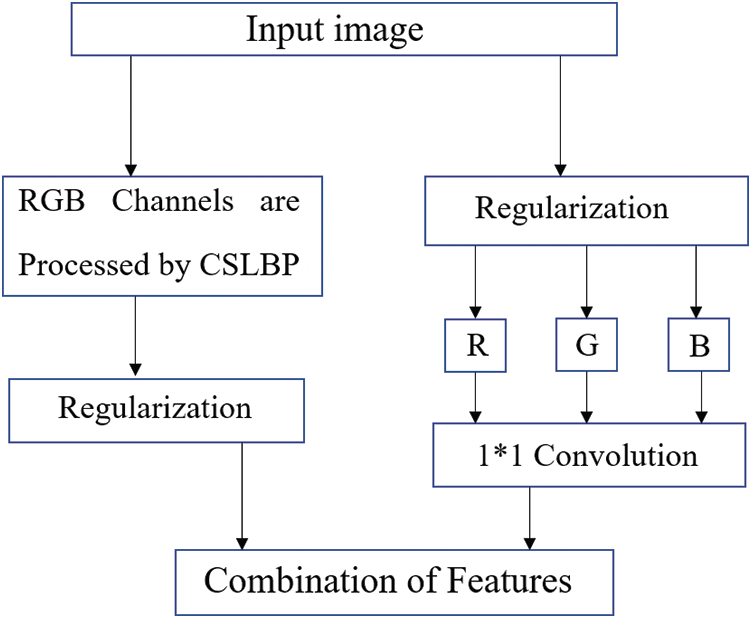Figure 5: Feature combination

The input is a RGB three-channel color image, assuming the image is m×n×3, expressed in the form of X=[R,G,B], where R, G, and B are matrices of size m×n. The characteristic image obtained after the input image is processed by CSLBP is Y=CSLPB(X)=[RCSLPB,GCSLPB,BCSLPB]. At the same time, the feature image obtained after the image is convolved Z=conv(X)=[Rconv,Gconv,Bconv], combining the two features, the image is [Y,Z]=[RCSLPB,GCSLPB,BCSLPB,Rconv,Gconv,Bconv].

The idea of this method is to combine the information of the original picture, which is proceeded by a local binary pattern with the information after unit convolution. This combination has two advantages: 1) The CSLBP value Y of the image and the feature information Z after convolution have different types of feature information. The combination of the two kinds of information makes the feature extraction of the convolutional neural network more sufficient. 2) Unit convolution processing of the original image can reduce its noise, which is more conducive to the effective feature extraction of the image.

4  Experimental Results and Analysis

In order to research the result of estimation of face age from face images through the network framework designed in this article, the hardware environment selected for the experiment was a computer Dell R730 server, the development platform was a Windows 2012 R2 server, and the development environment was Python 3.6; tensorflow-gpu 1.9.0; PyCharm community 2018.3.1.

This experiment verified the effect of face age estimation after processing face images with CSLBP operator. During the training process of the experiment, the face area of the face image in the dataset was adjusted to a resolution of 64 × 64. The experimental network model was mainly implemented with Keras, and the network model was optimized by Adam. SSR-Net used three stages, of which s1 = s2 = s3, and Adam optimized network parameters of 90 epochs. The initial learning rate of the experiment in the parameter setting was lr = 0.001, which was reduced by a factor of 0.1 every 30 epochs. The batch size of the IMDB data set was 128, and the batch size of the MORPH 2 data set was 50.

4.1 Data Set

The data sets used in this article included three data sets: MORPH 2, IMDB, and WIKI. Among them, the age statistics of the MORPH 2 data set are illustrated in Fig. 6a, which contains 55,134 images from 13,618 people, the age range is 16–77 years old, and the data set annotation type is the age value; the IMDB and WIKI data sets are shown in Figs. 6b and 6c, respectively, and contain 523,051 face images with age and gender annotations. The age range is 0–100 years old and belongs to the true age data set. The age distribution of the data set used is shown in Fig. 6. It can be seen that most images belong to people in the range of 20–40 years old.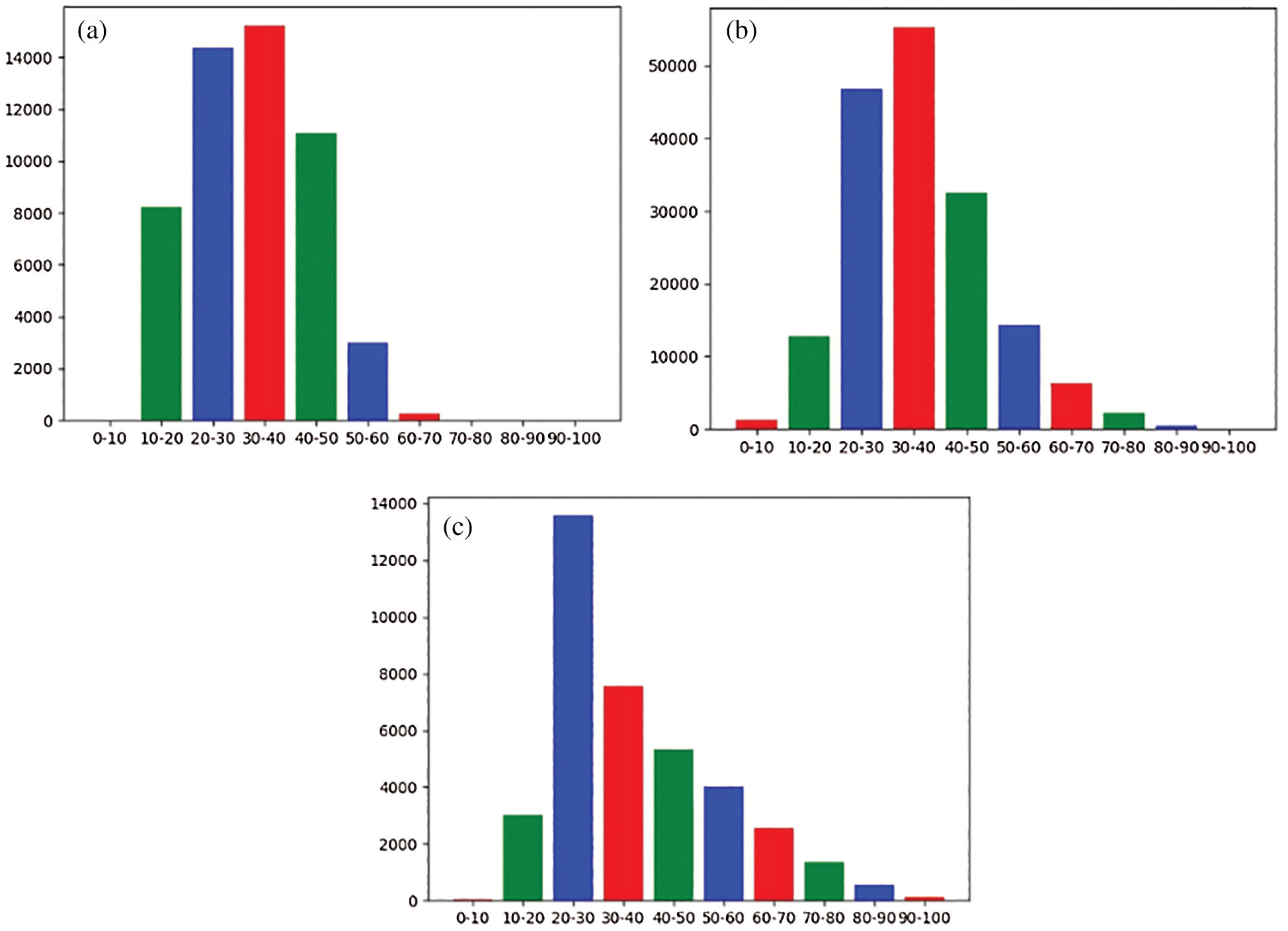Figure 6: Statistical histogram of the data sets. (a) MORPH 2 data set, (b) IMDB data set, (c) WIKI data set

4.2 Evaluation Standard

In this paper, the average absolute error (MAE) value was used as the measurement standard for the estimation of face age. The average absolute error refers to the average of the true value of age and the absolute error of predicted age. Therefore, the smaller the value of MAE, the more accurate the estimation is. Its expression is:

MAE=1Ni=1N|sk~sk| (6)

where sk is the true age of the sample, sk~ is the age predicted by the network, and N is the total number of interval samples.

4.3 Experimental Results and Analysis

In order to verify the effectiveness and efficiency of the method and model proposed in this article, the comparative experiment of this experiment was divided into four groups: the first group input the original face image into the network model; the second group was the feature image after CSLBP processing input into the network model; the third group combined the feature images after LBP processing with the feature images of the original face image and then input them into the network model; the fourth group of experiments replaced the LBP processing in the previous group with CSLBP. After the face image was processed by the CSLBP operator, a feature image was obtained. The feature image had the texture information of the face image and also reduced the impact of the image due to illumination. The four groups of experiments were carried out using the MORPH 2 data set. The comparison results are shown in Tab. 1. It can be seen that the recognition rate of face authentication obtained by using only CSLBP feature image as the input of the network is lower than the recognition rate obtained by using the original RGB image as there is information loss when an RGB image is converted into a CSLBP feature image. At the same time, when the LBP method is used for processing and the CSLBP method is used as a comparison, the processing time with LBP method is longer while the accuracy is not much different. However, CSLBP feature images have features that RGB images do not have, so the recognition rate of face authentication obtained by combining RGB images and CSLBP feature images is higher than the recognition rate obtained by using RGB images or CSLBP feature images alone. This method has a disadvantage in that adding CSLBP information will increase network training time because extracting CSLBP features takes time.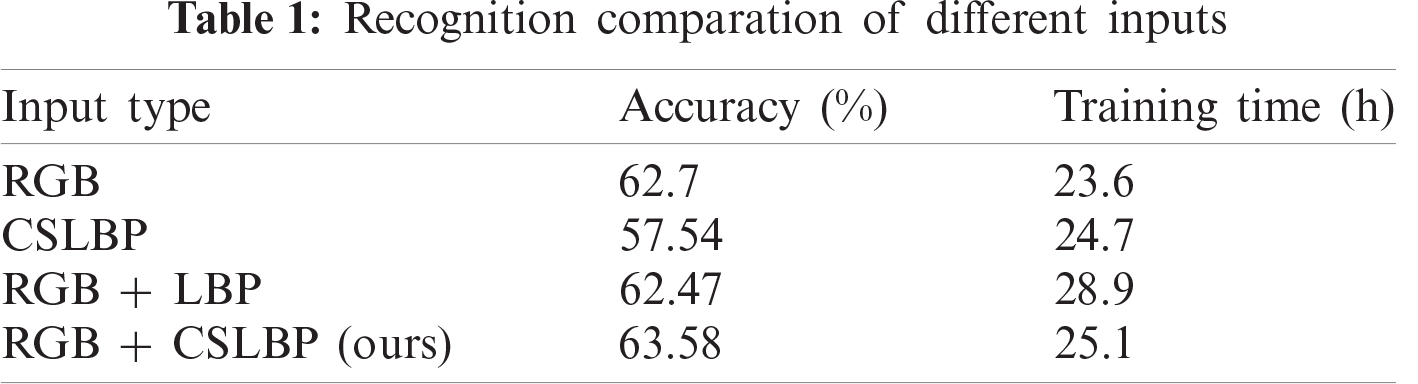Figs. 7a7c are the MAE change curves of the training set and the validation set in the IMDB, WIKI, and MORPH 2 datasets, respectively. It can be seen from the figure that the average absolute error after 30 iterations tends to be stable, and there is basically no obvious change after 80 iterations. The blue curve in MAE represents the change in training error, and the orange curve represents the change in verification error. In the figure, it can be clearly observed that the two curves are close after a certain number of iterations, which means that the model obtained from the training data can be better applied to the verification data. Therefore, SSR-Net is less affected by overfitting on the three data sets.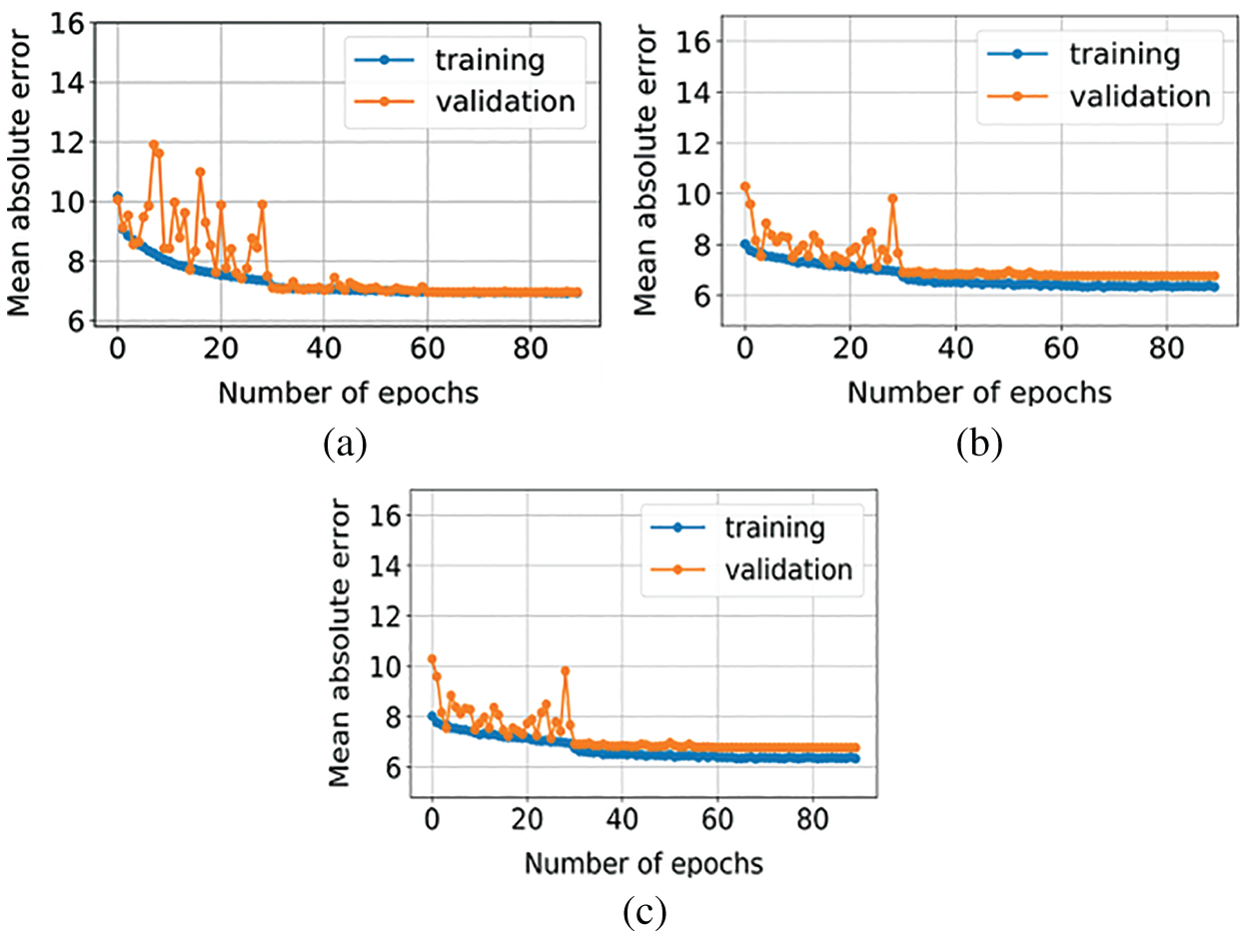Figure 7: MAE change curve. (a) IMDB, (b) WIKI, (c) MORPH 2

In the problem of estimating the true age from a single face image, we get a set of training face images X={xnn=1N}, and the real age ynY for each image xn, where N is the number of images and Y is the interval of ages. The goal is to find a function F that predicts y~=F(x) as the age for a given image x. For training, we search for the function F by minimizing the mean absolute error (MAE) between the predicted and the real ages, it is defined as below.

J(x)=1Nn=1N|yn~yn| (7)

Referring to Eq. (7), yn~=F(xn) is the predicted age for training image xn. For training under the data set, the loss changes of the test set and the verification set are shown in Fig. 8. It can be seen from the figure that the loss gradually decreases with the increase in the number of iterations and stabilizes at the end. Both the validation set and the test set can reduce the loss under the average loss function and also obtain a better training effect.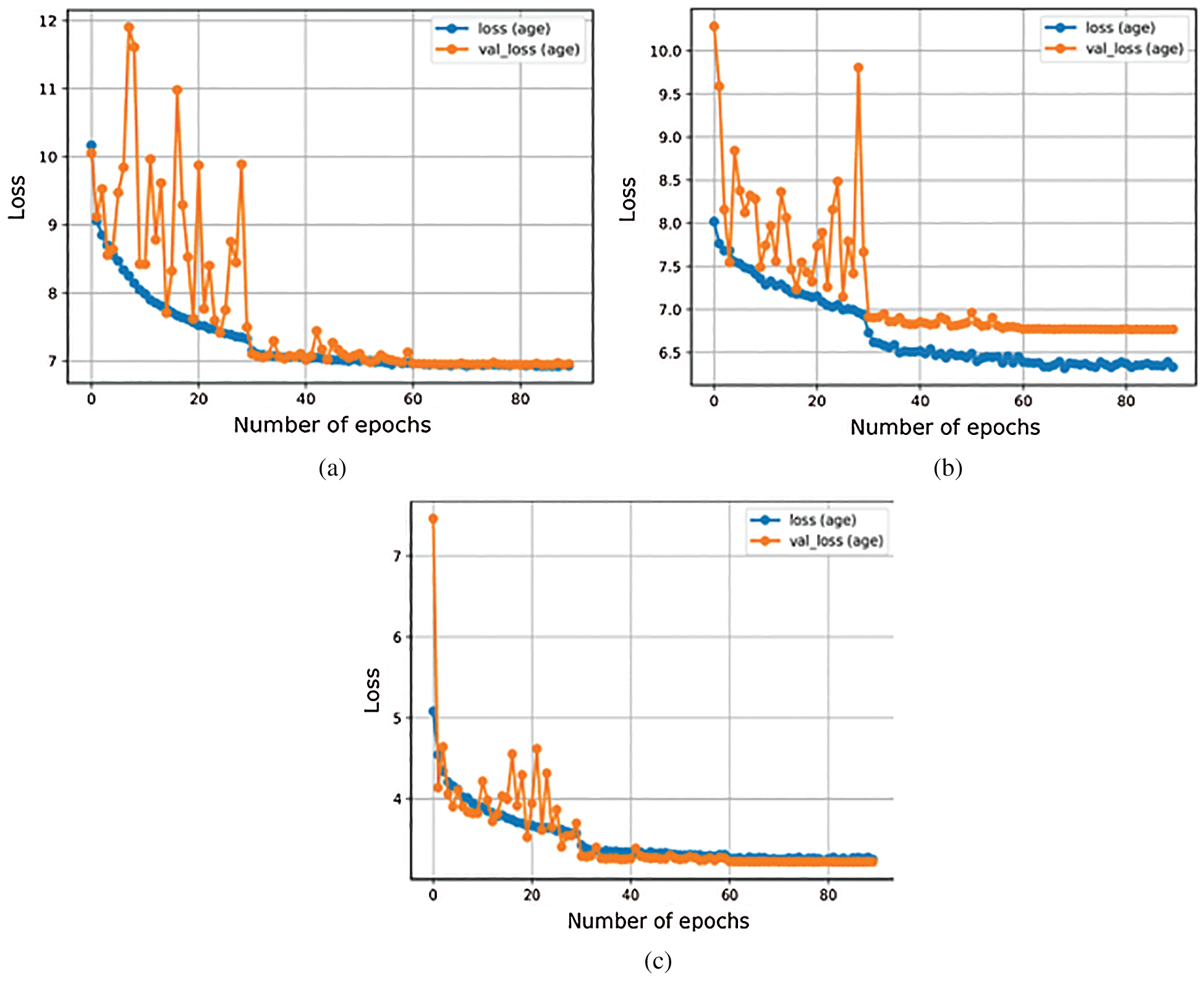Figure 8: Loss change curve. (a) IMDB, (b) WIKI, (c) MORPH 2

In order to perform a more detailed analysis of the performance of the proposed model, we also applied a confusion matrix to the model. Fig. 9 shows the confusion matrix for the age range. It can be clearly seen that on the main diagonal of the matrix, most of the cases are predicted to be the true labels of the category. It is worth noting that the largest false alarm rate in the age range classification model corresponds to images belonging to the 40–50 age group, which are mistaken for 50–60 years old.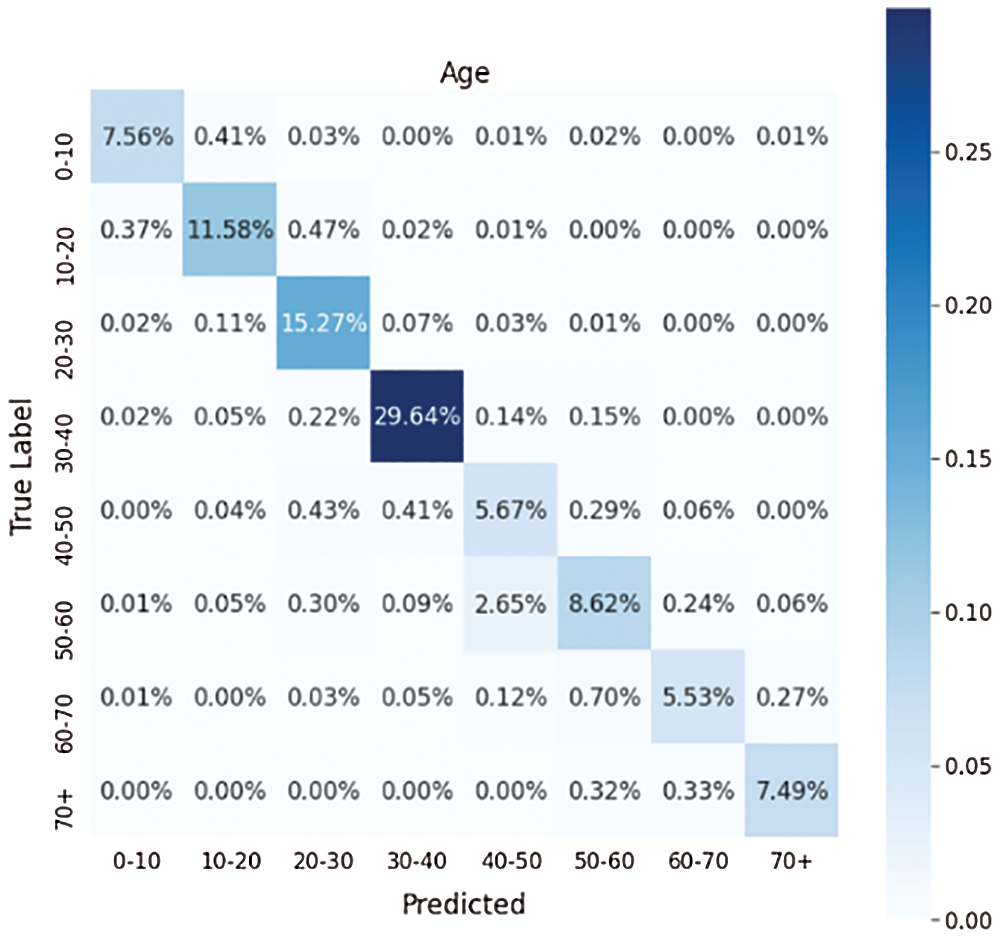Figure 9: Confusion matrix for age estimation

5  Conclusion

We presented a face age estimation method based on CSLBP and lightweight convolutional neural network in this work. A combination of CSLBP operator with the SSR network was proposed for the first time, and CSLBP was used to process the original face image. This processing method could effectively reduce the impact of the face image on the light change. The CSLBP processed feature image combined with the original face image was then input to the SSR-Net to perform feature extraction and age estimation. The model in this paper is smaller than the neural network model explored by its predecessors, and the processing speed will be further improved when the network parameters are small, supporting its further development on mobile terminals. In this paper, four sets of comparative experiments illustrated that the improved method could improve the robustness of the model against the influence of illumination under the feature input after adding texture information while also improving estimation accuracy.

Funding Statement: This work was funded by the foundation of Liaoning Educational committee under the Grant No. 2019LNJC03.

Conflicts of Interest: The authors declare that they have no conflicts of interest to report regarding the present study.

References

1.  1.  C. M. Yan and C. Wang, “Development and application of convolutional neural network model,” Computer Science and Exploration, vol. 15, no. 1, pp. 27–46, 2020.
2.  2.  S. Zaghbani, N. Boujneh and M. S. Bouhlel, “Age estimation using deep learning,” Computers & Electrical Engineering, vol. 68, pp. 337–347, 2018.
3.  3.  K. Zhang, X. S. Wang, Y. R. Guo, Y. K. Su and Y. X. He, “A review of deep learning methods for face age estimation,” Journal of Image and Graphics, vol. 24, no. 8, pp. 1215–1230, 2019.
4.  4.  E. N. A. Hammond, S. Zhou, H. Cheng and Q. Liu, “Improving Juvenile age estimation based on facial landmark points and gravity moment,” Applied Sciences-Basel, vol. 10, no. 18, pp. 6227–6244, 2020.
5.  5.  W. Deng and X. Liang, “Face detection based on BP neural network and improved AdaBoost algorithm,” in Proc. Int. Conf. on Cloud Computing and Intelligence Systems 2018 5th IEEE, Nanjing, China, pp. 395–399, 2018.
6.  6.  V. Martin, R. Seguier, A. Porcheron and F. Morizot, “Face aging simulation with a new wrinkle oriented active appearance model,” Multimedia Tools and Applications, vol. 78, no. 5, pp. 6309–6327, 2018.
7.  7.  X. Geng, Z. H. Zhou and K. Smith-Miles, “Automatic age estimation based on facial aging patterns,” IEEE Transactions on Pattern Analysis and Machine Intelligence, vol. 29, no. 12, pp. 2234–2240, 2007.
8.  8.  M. Kang and M. Jung, “Low-dimensional manifold model for demosaicking from a RGBW color filter array,” Signal Image and Video Processing, vol. 14, no. 1, pp. 143–150, 2019.
9.  9.  Z. P. Ji, “Research on facial age estimation algorithm based on video,” Ph.D. dissertation. Beijing Jiao tong University, China, 2019.
10. 10. J. Tang, Q. Su, B. Su, S. Fong, W. Cao et al., “Parallel ensemble learning of convolutional neural networks and local binary patterns for face recognition,” Computer Methods Programs in Biomed, vol. 197, no. 12, pp. 105622–105629, 2020.
11. 11. Z. Yang and H. Ai, “Demographic classification with local binary patterns,” in Proc. Int. Conf. on Biometrics, Berlin, Heidelberg, Springer, pp. 464–473, 2007.
12. 12. D. Yi, Z. Lei and S. Z. Li, “Age estimation by multi-scale convolutional network,” in Proc. Asian Conf. Computer Vision, Cham, Springer, pp. 144–158, 2014.
13. 13. K. Li, J. Xing, C. Su, W. Hu, Y. Zhang, et al., “Deep cost-sensitive and order-preserving feature learning for cross-population age estimation,” in Proc. Computer Vision and Pattern Recognition, Salt Lake City, UT, USA, pp. 399–408, 2018.
14. 14. J. Xing, K. Li, W. Hu, C. Yuan and H. Ling, “Diagnosing deep learning models for high accuracy age estimation from a single image,” Pattern Recognition, vol. 66, no. 11, pp. 106–116, 2017.
15. 15. Y. Wen, K. Zhang, Z. Li and Y. Qiao, “A discriminative feature learning approach for deep face recognition,” in Proc. Computer Vision–ECCV, 2016 14th European Conf., Amsterdam, The Netherlands, pp. 499–515, 2016.
16. 16. C. Zegedy, W. Liu, Y. Jia, P. Sermanet, S. Reed et al., “Going deeper with convolutions,” in Proc. Computer Vision and Pattern Recognition, Boston, America, pp. 1–9, 2015.
17. 17. D. Gyawali, P. Pokharel, A. Chauhan and S. C. Shakya, “Age range estimation using MTCNN and VGG-Face model,” in Proc. 11th 2020 Int. Conf. on Computing, Proc.: Communication and Networking Technologies, West Bengal, India, pp. 1–6, 2020.
18. 18. K. He, X. Zhang, S. Ren and J. Sun, “Deep residual learning for image recognition,” in Proc. 2016 IEEE Conf. on Computer Vision and Pattern Recognition, Las Vegas, NV, USA, pp. 770–778, 2016.
19. 19. R. Angulu, J. R. Tapamo and A. O. Adewumi, “Age estimation via face images: A survey,” EURASIP Journal on Image and Video Processing, vol. 2018, no. 1, pp. 1–35, 2018.
20. 20. L. L. Zhu, F. Chen and C. Gao, “Improvement of face detection algorithm based on lightweight convolutional neural network,” in Proc. 2020 IEEE 6th Int. Conf. on Computer and Communications, Chengdu, China, pp. 1191–1197, 2020.
21. 21. J. Li and L. He, “Age estimation of face images based on deep learning,” Modern Information Technology, vol. 3, no. 18, pp. 40–42, 2019.
22. 22. Z. Zhu, J. Li, Y. Hu and X. Deng, “Research on age estimation algorithm based on structured sparsity,” International Journal of Pattern Recognition and Artificial Intelligence, vol. 33, no. 6, pp. 1956006–1956025, 2019.
23. 23. H. Xiang, Y. Zhao, Y. Yuan, G. Zhang and X. Hu, “Lightweight fully convolutional network for license plate detection,” Optik, vol. 178, no. 15, pp. 1185–1194, 2019.
24. 24. T. Y. Yang, Y. H. Huang, Y. Y. Lin, P. C. Hsiu and Y. Y. Chuang, “SSR-Net: A compact soft stagewise regression network for age estimation,” in Proc. Twenty-Seventh Int. Joint Conf. on Artificial Intelligence, Stockholm, Sweden, pp. 1–7, 2018.
25. 25. S. k. Xu, L. Qiu, N. Li and L. Shi, “Pedestrian detection based on HOG-CSLBP and YOLOv2,” Computer Engineering and Design, vol. 40, no. 10, pp. 2964–2968, 2019.
26. 26. T. Li, Y. Wang, Y. Chen, Y. J. Ren, L. Wang et al., “A face recognition algorithm based on LBP-EHMM,” Journal on Artificial Intelligence, vol. 1, no. 2, pp. 61–68, 2019.This work is licensed under a Creative Commons Attribution 4.0 International License, which permits unrestricted use, distribution, and reproduction in any medium, provided the original work is properly cited.